+

# Introduction to Exponents

##### Rating:
(1)
• (0)
• (0)
• (1)
• (0)
• (0)
Author: Sophia Tutorial
##### Description:

Identify the correct evaluation of an expression with exponents.

(more)
Tutorial
what's covered
1. Introduction to Exponents
1. Exponents of 2 and 3
2. Exponents 1 and 0

# 1. Introduction to Exponents

An exponent is repeated multiplication. This means that if we have the same number used in multiplication several times, we can express this using an exponent. Let's take a look at an example: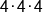We see the number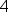three times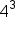Expressed using an exponent

Let's examine the relationship between the 4 and the 3. We refer to 4 as the base of the expression. This is the number that is being multiplied. 3 is called the exponent, and it tells us how many times to use the base in a chain of multiplication. So we interpret 4 cubed as repeated multiplication: using 4 as the base in a chain of multiplication 3 times.

How do we read expressions with exponents? 4 cubed is read as "4 to the 3rd power." Here are some other ways to say the same thing:to the power of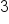raised to therdraised to therd powerraised to the power ofSo common language includes words such as "power" and "raised" and we read the base number as a normal standard number, but the exponent could be read as an ordinal number (first, second, third, etc.)

Next we are going to talk about some common exponents: 2 and 3.

1a. Exponents of 2 and 3

When a base is raised to the power of 2, a common way of talking about something to the power of two is using the term "squared." Think about the area of a square. A square has side lengths that are equal to each other, and we multiply them together to find the area. So the side length squared gives us the area of the square.

When a base is raised to the power of 3, we commonly say that the base is "cubed." Like squaring and area, we can think about the volume of a cube. A cube has side lengths that are equal in measure, and we multiply the dimensions to find the volume. So the side length cubed gives us the volume of a cube.

big idea
Any base raised to the exponent 2 is called squaring the base. Any base raised to the exponent 3 is called cubing the base.

1b. Exponents of 1 and 0

Now let's talk about two other special exponents: one and zero. Recall that the exponent tells us how many times to use the base in a chain of multiplication. So if the exponent is 1, the expression simply equals the base, no matter what the base is.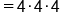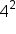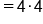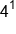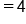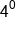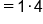What if the exponent is zero. A common mistake is to think that anything raised to the power of zero is zero, but this is not correct. Let's approach a zero exponent by writing another expression with an exponent, and working our way down to an exponent of zero.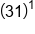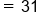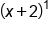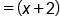big idea
Any number, variable, or expression raised to the power of 1 remains the same. Any number, variable, or expression raised to the power of zero equals 1.

summary
Exponents are used to denote how many times a number is being multiplied by itself or repeated multiplication. In the form b to the power of x, the b is the number that is being multiplied, which we call our base. The x is the exponent, which tells us how many times we're multiplying by that base.

Common cases are exponents of 2 and 3. Any base raised to the exponent 2 is called squaring the base. Any base raised to the exponent 3 is called cubing the base. There are also special cases involving exponents; exponents of 1 and 0. Any number, variable, or expression raised to the power of 1 remains the same. Any number, variable, or expression raised to the power of zero equals 1.

Rating Header